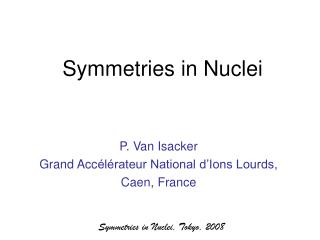DownloadDownload PresentationSymmetries in Nuclei

# Symmetries in Nuclei

Télécharger la présentation## Symmetries in Nuclei

- - - - - - - - - - - - - - - - - - - - - - - - - - - E N D - - - - - - - - - - - - - - - - - - - - - - - - - - -
##### Presentation Transcript

1. Symmetries in Nuclei • P. Van Isacker • Grand Accélérateur National d’Ions Lourds, • Caen, France Symmetries in Nuclei, Tokyo, 2008

2. Symmetries in Nuclei • Symmetry and its mathematical description • The role of symmetry in physics • Symmetries of the nuclear shell model • Symmetries of the interacting boson model Symmetries in Nuclei, Tokyo, 2008

3. What is symmetry? • Oxford Dictionary of English: “(beauty resulting from the) right correspondence of parts; quality of harmony or balance (in size, design) between parts” • Examples: disposition of a French garden; harmony of themes in a symphony. Symmetries in Nuclei, Tokyo, 2008

4. Etymology • Ancient Greek roots: • “sun” means “with, together” • “metron” means “measure” • For the ancient Greeks symmetry was closely related to harmony, beauty and unity. • Plato (Timaeus): Regular polyhedra are afforded a central place in the doctrine of natural elements. • Aristotle: “The chief forms of beauty are orderly arrangement [taxis], proportion [symmetria] and definiteness [horismenon].” Symmetries in Nuclei, Tokyo, 2008

5. Origin • For the ancient Greeks symmetry implied the notion of commensurability and proportion. • 17th century: Symmetry starts to imply also an relation of equality of elements that are opposed (eg. between left and right). • 19th century: Definition of symmetry via the notion of invariance under transformations such as translations, rotations, reflections. Introduction of the notion of a group of transformations. Symmetries in Nuclei, Tokyo, 2008

6. Symmetries around us • Bilateral symmetry. • Translational symmetry. • Rotational symmetries. • Reflection-rotation symmetry. • Glide-reflection symmetry. • Scale invariance. • Conformal invariance. • Temporal symmetries. Symmetries in Nuclei, Tokyo, 2008

7. Bilateral symmetry • Object with two halves that are each others mirror image. • Most widespread symmetry in Nature: (wo)man, animals, insects, flowers… Symmetries in Nuclei, Tokyo, 2008

8. Palindromes • Word equivalent of objects with bilateral symmetry, invented by Sotades the Obscene of Maronea. • Examples: • Napoleon: “Able was I ere I saw Elba.” • In Dutch (kreeftzin or ‘lobster sentence’): “Nelli plaatst op ‘n parterretrap ‘n pot staalpillen.” • About 10% of DNA letters form palindromic sequences, essential in the fight against mutations. Symmetries in Nuclei, Tokyo, 2008

9. Bilateral symmetry • Man is conditioned by bilateral symmetry (cfr. Rorschach test). • Symmetry plays a crucial role in mate selection. Symmetries in Nuclei, Tokyo, 2008

10. Rotational symmetry • Object that transforms into itself after rotation over a given angle. • Two types occur frequently in Nature: • Discrete rotations over 2/n where n is the order of the rotation • Continuous rotations: axial or spherical Symmetries in Nuclei, Tokyo, 2008

11. Discrete rotations Order 3: clover Order 5: starfish Order 7: algae Order 9: centriole Symmetries in Nuclei, Tokyo, 2008

12. Continuous rotations Axial symmetry Spherical symmetry Soap bubble Heliozoaire Symmetries in Nuclei, Tokyo, 2008